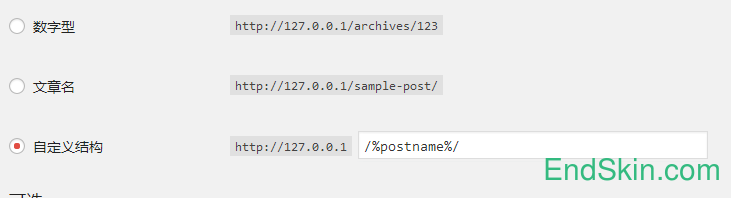WordPress 最终完美解决文章 固定链接ID 不连续的问题方案

批量设置

```function Bing_post_id_continuous_query(){
set_time_limit( 0 );
remove_action( 'publish_post', 'Bing_post_id_continuous' );
query_posts( 'posts_per_page=-1' );
\$arr = array();
while( have_posts() ){
the_post();
\$post_id = \$GLOBALS['post']->ID;
\$arr[] = \$post_id;
}
wp_reset_query();
\$arr = array_reverse( \$arr );
\$i = 1;
foreach( \$arr as \$post_id ){
wp_update_post( array(
'ID' => \$post_id,
'post_name' => \$i++
) );
}
}
if( \$_GET['post_id_continuous_query'] == 'yes' && current_user_can( 'level_10' ) ) add_action( 'init', 'Bing_post_id_continuous_query' );```

http://example.com?post_id_continuous_query=yes

新文章自动设置

```function Bing_post_id_continuous( \$id, \$post, \$update ){
if( \$update || \$post->post_status != 'publish' ) return;
\$action = 'save_post_post';
\$func = 'Bing_post_id_continuous';
remove_action( \$action, \$func, 10 );
wp_update_post( array(
'ID' => \$id,
'post_name' => wp_count_posts()->publish + 1
));
add_action( \$action, \$func, 10, 3 );
}
add_action( 'save_post_post', 'Bing_post_id_continuous', 10, 3 );```

2014-07-31 更新：让更新文章时不重新计算别名。

修改固定链接错乱

```function Bing_post_name_id(){
query_posts( 'posts_per_page=-1' );
while( have_posts() ){
the_post();
\$post_id = \$GLOBALS['post']->ID;
wp_update_post( array(
'ID' => \$post_id,
'post_name' => \$post_id
) );
}
wp_reset_query();
}
if( \$_GET['post_name_id'] == 'yes' ) add_action( 'init', 'Bing_post_name_id' );```

http://example.com?post_name_id=yes

TA的作品

Q Q 登 录 微 博 登 录

Q Q 登 录 微 博 登 录